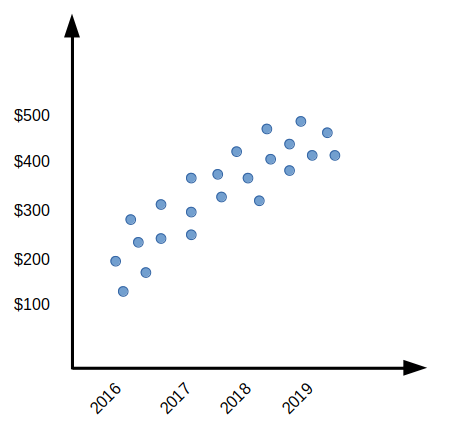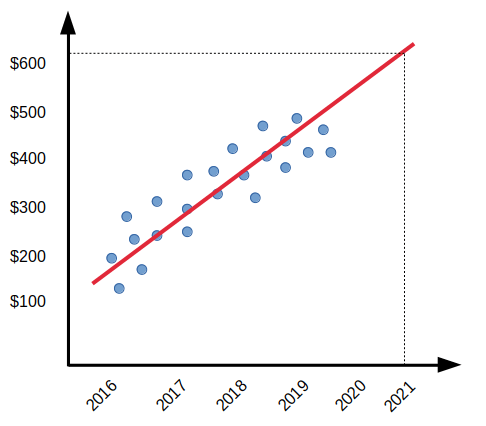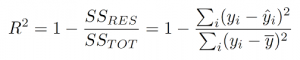# Linear Regression in Machine Learning

By Learnbay Category Machine Learning Reading time 6-8 mins Published on Dec 30, 2020

### What is Regression?

In statistical modeling, regression analysis is a statistical process for estimating the relationships among variables. It includes many techniques for modeling and analyzing several variables when the focus is on the relationship between a dependent variable and one or more independent variables (or ‘predictors’). Regression is a predictive modeling analysis technique. It estimates a relationship between the dependent and an independent variable.

Use of Regression:

• Determine the strength of predictors.
• Forecasting an effect.
• Trend forecasting.

Linear Regression:

Linear regression is a basic and commonly used type of predictive analysis. The overall idea of regression is to examine two things, it does a set of predictor variables do a good job in predicting an outcome (dependent) variable? in Which variables, in particular, are significant predictors of the outcome variable, and in what way do they–indicated by the magnitude and sign of the beta estimates–impact the outcome variable? These regression estimates are used to explain the relationship between one dependent variable and one or more independent variables. The simplest form of the regression equation with one dependent and one independent variable is defined by the formula y = c + b*x, where y = estimated dependent variable score, c = constant, b = regression coefficient, and x = score on the independent variable.

Linear Regression Selection Criteria:

1. Classifiaction & Regression capabalities.
2. Data quality.
3. Computational complexity.
4. Comprehensive & transport.

When will we use Linear Regression?

• Evaluating trends & sales estimates.
• Analyzing the impact of price changes.
• Assessment of risk in financial services and insurance domain.for example, a group of creative Tech enthusiasts started a company in Silicon Valley. This start-up — called Banana — is so innovative that it has been growing constantly since 2016. You, the wealthy investor, would like to know whether to put your money on Banana’s success in the next year or not. Let’s assume that you don’t want to risk a lot of money, especially since the stakes are high in Silicon Valley. So you decide to buy a few shares, instead of investing in a big portion of the company.

Well, you can definitely see the trend. Banana is growing like crazy, kicking up their stock price from 100 dollars to 500 in just three years. You only care about how the price is going to be like in the year 2021 because you want to give your investment some time to blossom along with the company. Optimistically speaking, it looks like you will be growing your money in the upcoming years. The trend is likely not to go through a sudden, drastic change. This leads to you hypothesizing that the stock price will fall somewhere above the \$500 indicator.

Here’s an interesting thought. Based on the stock price records of the last couple of years you were able to predict what the stock price is going to be like. You were able to infer the range of the new stock price (that doesn’t exist on the plot) for a year that we don’t have data for (the year 2021). Well — kinda.

What you just did is infer your model (that head of yours) to generalize — predict the y-value for an x-value that is not even in your knowledge. However, this is not accurate in any way. You couldn’t specify what exactly is the stock price most likely going to be. For all you know, it is probably going to be above 500 dollars.

Here is where Linear Regression (LR) comes into play. The essence of LR is to find the line that best fits the data points on the plot so that we can, more or less, know exactly where the stock price is likely to fall in the year 2021.Let’s examine the LR-generated line (in red) above, by looking at the importance of it. It looks like, with just a little modification, we were able to realize that Banana’s stock price is likely to be worth a little bit higher than \$600 by the year 2021. Obviously, this is an oversimplified example. However, the process stays the same. Linear Regression as an algorithm relies on the concept of lowering the cost to maximize the performance. We will examine this concept, and how we got the red line on the plot next.

Finding the best fit line:

To check the goodness of fit we use the R-squared method.

What is the R-squared method?

R-squared value is a statistical measure of how close the data to the fitted linear regression line. It is also known as COD(coefficient of determination), or the coefficient of multiple determination.## What are overfitting and underfitting?

Overfitting: Good performance on the training data, poor generalization to other data.

Underfitting: Poor performance on the training data & poor generalization to other data.

Linear Regression with python:

1.Importing required libraries:

import numpy as np

from sklearn.linear_model import LinearRegression

1. Provide data:

x = np.array([5, 15, 25, 35, 45, 55]).reshape((-1, 1))

y = np.array([5, 20, 14, 32, 22, 38])

print(x)

print(y)

Output:

print(x)

[









]

print(y)

[ 5 20 14 32 22 38]

1. Create a model and fit it:

model = LinearRegression().fit(x, y)

1. Get Result:

r_sq = model.score(x, y)

print('coefficient of determination:', r_sq)

coefficient of determination: 0.715875613747954

1. Predict response:

y_pred = model.predict(x)

print('predicted response:', y_pred, sep='\n')

predicted response:

[ 8.33333333 13.73333333 19.13333333 24.53333333 29.93333333 35.33333333]

Learnbay provides industry accredited data science courses in Bangalore. We understand the conjugation of technology in the field of Data science hence we offer significant courses like Machine Learning, Tensor Flow, IBM Watson, Google Cloud platform, Tableau, Hadoop, time series, R and Python. With authentic real-time industry projects. Students will be efficient by being certified by IBM. Around hundreds of students are placed in promising companies for data science roles. Choosing Learnbay you will reach the most aspiring job of present and future.

Learnbay data science course covers Data Science with Python, Artificial Intelligence with Python, Deep Learning using Tensor-Flow. These topics are covered and co-developed with IBM.